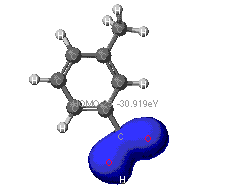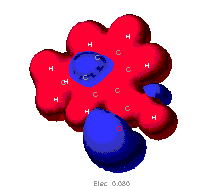# Theoretical Calculations of Properties of m-Toluic Acid

by Eric Kiefer

### Types of calculations performed

Determination of any molecular property is done by making a measurement in the lab. Due to recent development of high-speed computers, quantum mechanics have been made much more practical. As a result, approximate values for properties such as dipole moment can be found before doing any experiment.

At the most basic level, quantum mechanics is a set of mathematical equations used to position all of the atoms and electrons so that the molecule has the lowest possible energy. In contrast with molecular mechanics, quantum mechanics does not find a geometry that spaces atoms equally. Instead, quantum mechanics will find a geometry resulting in the lowest energy. The process of minimizing the energy of the molecule is actually the boring, repetitive part of quantum mechanics; it is accomplished by initially choosing a reasonable structure (the result of molecular mechanics) and then moving one particle at a time until the energy of the system is as low as possible.

All of the following calculations have been made using quantum mechanics. Because these computations are very time consuming and very repetitive, a software package called CACHE Workshop was used to do the mathematics. Included in this package are three programs named after the respective calculation it performs: Extended Huckel, ZINDO, and MOPAC. Although they all perform quantum mechanics, they each make different assumptions. For Extended Huckel, it assumes that the structure of the molecule is determined by molecular mechanics. This assumption, among others, works well for aromatic or conjugated systems. Both ZINDO and MOPAC are semi-empirical in that they incorporate results from spectroscopy. ZINDO calculations are good for predicting electronic spectra, and MOPAC works the best for any general molecule since it makes the fewest approximations in doing the math.

### Results of geometry optimization at various levels of theory

The classic ball-and-stick model for each level of theory is given below. Feel free to click on these molecules and move them around as you please. After examination, you should find the structures to be very similar; this should not be a surprise as these are relatively simple molecules.

The structure on the left was determined without quantum mechanics. It was determined by treating each bond as a very stiff spring and each atom as a ball. Using this model, the lowest energy structure is the one in which all of the atoms are spaced as far away from each other as possible.

The middle structure and the right structure were determined after quantum mechanics. For these structures, atoms and bonds were not treated as balls and sticks. Rather, they were treated as charged particles. The subatomic particles were tweaked a little at a time until the lowest possible energy was found.
 Molecular Mechanics ZINDO MOPAC

### The molecular orbitals that provide the primary bonding

Bonds are usually thought of as shared electrons between atoms. This idea can be deceptive if you limit yourself to just s-orbitals, p-orbitals and etc. What you see below are actually a bunch of orbitals added or subtracted together. It turns out that lower energy--and more stable--bonds can be formed using this method; below are a just a few of the bonding orbitals that exist. In examining these molecules, it is refreshing to see a couple familiar orbitals predicted by quantum mechanics. The ring shaped orbital in the middle model corresponds to a sigma bonding network. As the third diagram illustrates, strange bonding and even anti-bonding can also occur.### The highest occupied (HOMO) and lowest unoccupied (LUMO) molecular orbitals

These orbitals are the most involved in chemical reactions since they can most easily lose or gain electrons. The HOMO is the orbital in which there are "valence shell electrons." If this orbital is not filled, an additional electron from its surroundings is allowed to join the molecule in this orbital. Otherwise, if the HOMO is full, the next place that the electron can enter is in the orbital specified in the LUMO. All of the molecular orbitals shown on this page are for the lowest energy geometry of the molecule.  The HOMO is determined by putting pairs of electrons in the molecular orbitals starting with the lowest energy orbital, just as the ground state configuration of an atom is determined.These are the HOMO and LUMO generated by Extended Huckel calculations.These are the HOMO and LUMO calculated using ZINDO.These are the HOMO and LUMO calculated using MOPAC.

Note that each level gives a slightly different molecular orbital for the HOMO and the LUMO. Chances are that none of these are exactly correct because of the starting assumptions used.

### Electron density/distribution maps

Electron denisty reveals where electrons are in the molecule. Taking all of the orbitals into account, the three different methods reveal where electrons are most likely located at any given moment. Lighter colors indicate a higher electron density while the purple and te dark grey gives low electron density. Interestingly, each model gives a different description. As one can see, Extended Huckel and ZINDO show a high density around the oxygen atoms, whereas MOPAC shows a high density of electrons about the conjugated ring. In any case, if there are places of high and low density of electrons within the same molecule, then the molecule is polar. This makes sense since electrons, which have a charge, are not spread evenly throughout the molecule; therefore, there must be two "poles," or two different regions of charge. Be careful and remember that high density on opposite sides of the molecule cancel out when calculating dipole moments.Extended Huckel ZINDO MOPAC

### Charge distribution maps

Without focusing on any particular atom, it is possible to see how charge is spread throughout the molecule. In the diagrams below, blue depicts negative charge and red depicts positive charge. It is comforting to see that oxygen atoms are negatively charged as one would expect without doing quantum mechanics. Additionally, notice the correlation between the electron density and charge distribution. A high electron density about the oxygen correlates to a negative charge around the oxygen. When this occurs and there is an unequal distribution of charge in a molecule, the molecule is polar. The more separated, distinct, and unbalanced this distribution is, the greater the polarity.Extended Huckel ZINDO MOPAC

### Partial atomic charges

Considering each atom of the molecule, the partial atomic charge is the charge that one atom experiences. Using MOPAC, the partial atomic charges were calculated. Graphically, the surface below makes sense since oxygen and the conjugated ring system are highly charged (the same color coding applies as above). Again, the charge on these atoms create an unsymmetric and uneven charge distribution which means that this molecule is polar.### Calculated dipole moments

The mathematical quantity used to determine the direction and magnitude of the overall charge separtion (this is a vector) is called the dipole moment. Essentially, this is the weighted average of the atomic charges in 3 dimensional space. This is the essential quantity used to determine the polarity of some molecule. The greater the dipole moment, the more polar the molecule.
 Model Used Calculation (mu/D) Extended Huckel 4.469 ZINDO 1.910 MOPAC 2.282 Actual--Experimentally Derived ?